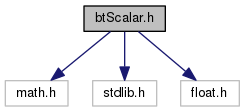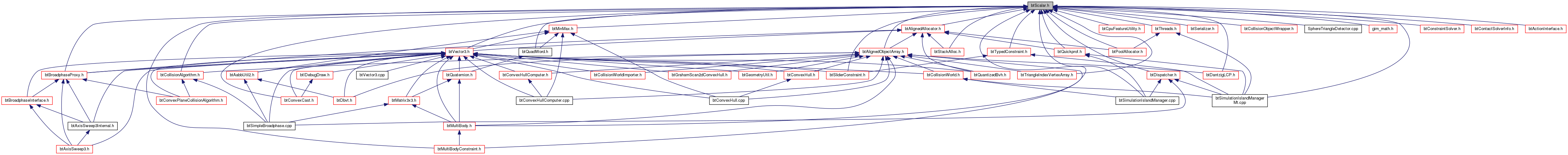Bullet Collision Detection & Physics Library
btScalar.h File Reference
`#include <math.h>`
`#include <stdlib.h>`
`#include <float.h>`
Include dependency graph for btScalar.h:This graph shows which files directly or indirectly include this file:Go to the source code of this file.

## Classes

struct  btTypedObject
rudimentary class to provide type info More...

## Macros

#define BT_BULLET_VERSION   288

#define BT_NOT_EMPTY_FILE

#define SIMD_FORCE_INLINE   __forceinline

#define ATTRIBUTE_ALIGNED16(a)   __declspec(align(16)) a

#define ATTRIBUTE_ALIGNED64(a)   __declspec(align(64)) a

#define ATTRIBUTE_ALIGNED128(a)   __declspec (align(128)) a

#define btAssert(x)

#define btFullAssert(x)

#define btLikely(_c)   _c

#define btUnlikely(_c)   _c

#define BT_LARGE_FLOAT   1e18f

#define BT_DECLARE_ALIGNED_ALLOCATOR()

#define SIMD_PI   btScalar(3.1415926535897932384626433832795029)

#define SIMD_2_PI   (btScalar(2.0) * SIMD_PI)

#define SIMD_HALF_PI   (SIMD_PI * btScalar(0.5))

#define SIMDSQRT12   btScalar(0.7071067811865475244008443621048490)

#define btRecipSqrt(x)   ((btScalar)(btScalar(1.0) / btSqrt(btScalar(x)))) /* reciprocal square root */

#define btRecip(x)   (btScalar(1.0) / btScalar(x))

#define SIMD_EPSILON   FLT_EPSILON

#define SIMD_INFINITY   FLT_MAX

#define BT_ONE   1.0f

#define BT_ZERO   0.0f

#define BT_TWO   2.0f

#define BT_HALF   0.5f

#define BT_DECLARE_HANDLE(name)

#define btFsels(a, b, c)   (btScalar) btFsel(a, b, c)

## Typedefs

typedef float btScalar
The btScalar type abstracts floating point numbers, to easily switch between double and single floating point precision. More...

## Functions

int btGetVersion ()

btScalar btSqrt (btScalar y)

btScalar btFabs (btScalar x)

btScalar btCos (btScalar x)

btScalar btSin (btScalar x)

btScalar btTan (btScalar x)

btScalar btAcos (btScalar x)

btScalar btAsin (btScalar x)

btScalar btAtan (btScalar x)

btScalar btAtan2 (btScalar x, btScalar y)

btScalar btExp (btScalar x)

btScalar btLog (btScalar x)

btScalar btPow (btScalar x, btScalar y)

btScalar btFmod (btScalar x, btScalar y)

btScalar btAtan2Fast (btScalar y, btScalar x)

bool btFuzzyZero (btScalar x)

bool btEqual (btScalar a, btScalar eps)

bool btGreaterEqual (btScalar a, btScalar eps)

int btIsNegative (btScalar x)

btScalar btDegrees (btScalar x)

btScalar btFsel (btScalar a, btScalar b, btScalar c)

bool btMachineIsLittleEndian ()

unsigned btSelect (unsigned condition, unsigned valueIfConditionNonZero, unsigned valueIfConditionZero)
btSelect avoids branches, which makes performance much better for consoles like Playstation 3 and XBox 360 Thanks Phil Knight. More...

int btSelect (unsigned condition, int valueIfConditionNonZero, int valueIfConditionZero)

float btSelect (unsigned condition, float valueIfConditionNonZero, float valueIfConditionZero)

template<typename T >
void btSwap (T &a, T &b)

unsigned btSwapEndian (unsigned val)

unsigned short btSwapEndian (unsigned short val)

unsigned btSwapEndian (int val)

unsigned short btSwapEndian (short val)

unsigned int btSwapEndianFloat (float d)
btSwapFloat uses using char pointers to swap the endianness More...

float btUnswapEndianFloat (unsigned int a)

void btSwapEndianDouble (double d, unsigned char *dst)

double btUnswapEndianDouble (const unsigned char *src)

template<typename T >
void btSetZero (T *a, int n)

btScalar btLargeDot (const btScalar *a, const btScalar *b, int n)

template<typename T >
T * btAlignPointer (T *unalignedPtr, size_t alignment)
align a pointer to the provided alignment, upwards More...

## Macro Definition Documentation

 #define ATTRIBUTE_ALIGNED128 ( a ) __declspec (align(128)) a

Definition at line 84 of file btScalar.h.

 #define ATTRIBUTE_ALIGNED16 ( a ) __declspec(align(16)) a

Definition at line 82 of file btScalar.h.

 #define ATTRIBUTE_ALIGNED64 ( a ) __declspec(align(64)) a

Definition at line 83 of file btScalar.h.

 #define BT_BULLET_VERSION   288

Definition at line 28 of file btScalar.h.

 #define BT_DECLARE_ALIGNED_ALLOCATOR ( )
Value:
SIMD_FORCE_INLINE void *operator new(size_t sizeInBytes) { return btAlignedAlloc(sizeInBytes, 16); } \
SIMD_FORCE_INLINE void operator delete(void *ptr) { btAlignedFree(ptr); } \
SIMD_FORCE_INLINE void *operator new(size_t, void *ptr) { return ptr; } \
SIMD_FORCE_INLINE void operator delete(void *, void *) {} \
SIMD_FORCE_INLINE void *operator new[](size_t sizeInBytes) { return btAlignedAlloc(sizeInBytes, 16); } \
SIMD_FORCE_INLINE void operator delete[](void *ptr) { btAlignedFree(ptr); } \
SIMD_FORCE_INLINE void *operator new[](size_t, void *ptr) { return ptr; } \
SIMD_FORCE_INLINE void operator delete[](void *, void *) {}
#define SIMD_FORCE_INLINE
Definition: btScalar.h:81
#define btAlignedFree(ptr)
#define btAlignedAlloc(size, alignment)

Definition at line 403 of file btScalar.h.

 #define BT_DECLARE_HANDLE ( name )
Value:
typedef struct name##__ \
{ \
int unused; \
} * name

Definition at line 569 of file btScalar.h.

 #define BT_HALF   0.5f

Definition at line 526 of file btScalar.h.

Definition at line 383 of file btScalar.h.

 #define BT_LARGE_FLOAT   1e18f

Definition at line 294 of file btScalar.h.

 #define BT_NOT_EMPTY_FILE

Definition at line 53 of file btScalar.h.

 #define BT_ONE   1.0f

Definition at line 523 of file btScalar.h.

 #define BT_TWO   2.0f

Definition at line 525 of file btScalar.h.

 #define BT_ZERO   0.0f

Definition at line 524 of file btScalar.h.

 #define btAssert ( x )

Definition at line 131 of file btScalar.h.

 #define btFsels ( a, b, c ) (btScalar) btFsel(a, b, c)

Definition at line 581 of file btScalar.h.

 #define btFullAssert ( x )

Definition at line 134 of file btScalar.h.

 #define btLikely ( _c ) _c

Definition at line 136 of file btScalar.h.

 #define btRecip ( x ) (btScalar(1.0) / btScalar(x))

Definition at line 511 of file btScalar.h.

 #define btRecipSqrt ( x ) ((btScalar)(btScalar(1.0) / btSqrt(btScalar(x)))) /* reciprocal square root */

Definition at line 510 of file btScalar.h.

 #define btUnlikely ( _c ) _c

Definition at line 137 of file btScalar.h.

 #define SIMD_2_PI   (btScalar(2.0) * SIMD_PI)

Definition at line 505 of file btScalar.h.

Definition at line 508 of file btScalar.h.

 #define SIMD_EPSILON   FLT_EPSILON

Definition at line 521 of file btScalar.h.

 #define SIMD_FORCE_INLINE   __forceinline

Definition at line 81 of file btScalar.h.

 #define SIMD_HALF_PI   (SIMD_PI * btScalar(0.5))

Definition at line 506 of file btScalar.h.

 #define SIMD_INFINITY   FLT_MAX

Definition at line 522 of file btScalar.h.

 #define SIMD_PI   btScalar(3.1415926535897932384626433832795029)

Definition at line 504 of file btScalar.h.

Definition at line 507 of file btScalar.h.

 #define SIMDSQRT12   btScalar(0.7071067811865475244008443621048490)

Definition at line 509 of file btScalar.h.

## Typedef Documentation

 typedef float btScalar

The btScalar type abstracts floating point numbers, to easily switch between double and single floating point precision.

Definition at line 292 of file btScalar.h.

## Function Documentation

 btScalar btAcos ( btScalar x )
inline

Definition at line 479 of file btScalar.h.

template<typename T >
 T* btAlignPointer ( T * unalignedPtr, size_t alignment )

align a pointer to the provided alignment, upwards

Definition at line 792 of file btScalar.h.

 btScalar btAsin ( btScalar x )
inline

Definition at line 487 of file btScalar.h.

 btScalar btAtan ( btScalar x )
inline

Definition at line 495 of file btScalar.h.

 btScalar btAtan2 ( btScalar x, btScalar y )
inline

Definition at line 496 of file btScalar.h.

 btScalar btAtan2Fast ( btScalar y, btScalar x )
inline

Definition at line 531 of file btScalar.h.

 btScalar btCos ( btScalar x )
inline

Definition at line 476 of file btScalar.h.

 btScalar btDegrees ( btScalar x )
inline

Definition at line 567 of file btScalar.h.

 bool btEqual ( btScalar a, btScalar eps )
inline

Definition at line 552 of file btScalar.h.

 btScalar btExp ( btScalar x )
inline

Definition at line 497 of file btScalar.h.

 btScalar btFabs ( btScalar x )
inline

Definition at line 475 of file btScalar.h.

 btScalar btFmod ( btScalar x, btScalar y )
inline

Definition at line 500 of file btScalar.h.

 btScalar btFsel ( btScalar a, btScalar b, btScalar c )
inline

Definition at line 576 of file btScalar.h.

 bool btFuzzyZero ( btScalar x )
inline

Definition at line 550 of file btScalar.h.

inline

Definition at line 384 of file btScalar.h.

 int btGetVersion ( )
inline

Definition at line 30 of file btScalar.h.

 bool btGreaterEqual ( btScalar a, btScalar eps )
inline

Definition at line 556 of file btScalar.h.

 int btIsNegative ( btScalar x )
inline

Definition at line 561 of file btScalar.h.

 btScalar btLargeDot ( const btScalar * a, const btScalar * b, int n )
inline

Definition at line 728 of file btScalar.h.

 btScalar btLog ( btScalar x )
inline

Definition at line 498 of file btScalar.h.

 bool btMachineIsLittleEndian ( )
inline

Definition at line 583 of file btScalar.h.

 btScalar btNormalizeAngle ( btScalar angleInRadians )
inline

Definition at line 759 of file btScalar.h.

 btScalar btPow ( btScalar x, btScalar y )
inline

Definition at line 499 of file btScalar.h.

 btScalar btRadians ( btScalar x )
inline

Definition at line 566 of file btScalar.h.

 unsigned btSelect ( unsigned condition, unsigned valueIfConditionNonZero, unsigned valueIfConditionZero )
inline

btSelect avoids branches, which makes performance much better for consoles like Playstation 3 and XBox 360 Thanks Phil Knight.

Definition at line 595 of file btScalar.h.

 int btSelect ( unsigned condition, int valueIfConditionNonZero, int valueIfConditionZero )
inline

Definition at line 605 of file btScalar.h.

 float btSelect ( unsigned condition, float valueIfConditionNonZero, float valueIfConditionZero )
inline

Definition at line 611 of file btScalar.h.

template<typename T >
 void btSetZero ( T * a, int n )
inline

Definition at line 717 of file btScalar.h.

 btScalar btSin ( btScalar x )
inline

Definition at line 477 of file btScalar.h.

 btScalar btSqrt ( btScalar y )
inline

Definition at line 444 of file btScalar.h.

template<typename T >
 void btSwap ( T & a, T & b )
inline

Definition at line 621 of file btScalar.h.

 unsigned btSwapEndian ( unsigned val )
inline

Definition at line 629 of file btScalar.h.

 unsigned short btSwapEndian ( unsigned short val )
inline

Definition at line 634 of file btScalar.h.

 unsigned btSwapEndian ( int val )
inline

Definition at line 639 of file btScalar.h.

 unsigned short btSwapEndian ( short val )
inline

Definition at line 644 of file btScalar.h.

 void btSwapEndianDouble ( double d, unsigned char * dst )
inline

Definition at line 684 of file btScalar.h.

 unsigned int btSwapEndianFloat ( float d )
inline

btSwapFloat uses using char pointers to swap the endianness

btSwapFloat/btSwapDouble will NOT return a float, because the machine might 'correct' invalid floating point values Not all values of sign/exponent/mantissa are valid floating point numbers according to IEEE 754. When a floating point unit is faced with an invalid value, it may actually change the value, or worse, throw an exception. In most systems, running user mode code, you wouldn't get an exception, but instead the hardware/os/runtime will 'fix' the number for you. so instead of returning a float/double, we return integer/long long integer

Definition at line 655 of file btScalar.h.

 btScalar btTan ( btScalar x )
inline

Definition at line 478 of file btScalar.h.

 double btUnswapEndianDouble ( const unsigned char * src )
inline

Definition at line 699 of file btScalar.h.

 float btUnswapEndianFloat ( unsigned int a )
inline

Definition at line 669 of file btScalar.h.